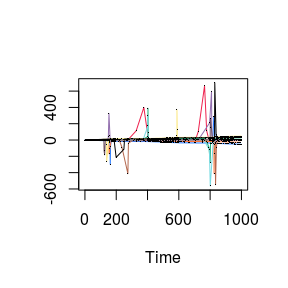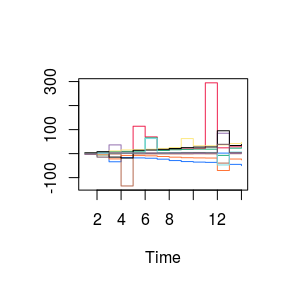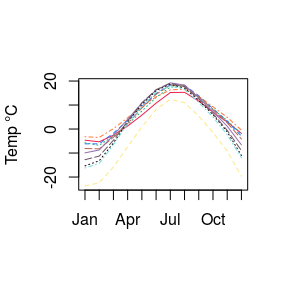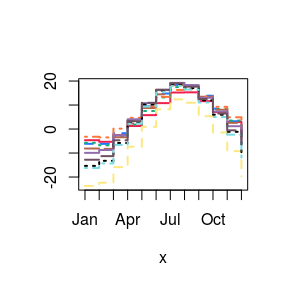# DrBats Data Simulation and Projection

## Overview

This document is part of the “DrBats” project whose goal is to implement exploratory statistical analysis on large sets of data with uncertainty. The idea is to visualize the results of the analysis in a way that explicitely illustrates the uncertainty in the data.

The “DrBats” project applies a Bayesian Latent Factor Model.

This project involves the following persons, listed in alphabetical order :

• Bénédicte Fontez (aut)

• Susan Holmes (aut)

• Gabrielle Weinrott (cre, aut)

## Main data simulation function

require(DrBats)
## Loading required package: DrBats
## Loading required package: rstan
## Loading required package: StanHeaders
## Loading required package: ggplot2
## rstan (Version 2.19.2, GitRev: 2e1f913d3ca3)
## For execution on a local, multicore CPU with excess RAM we recommend calling
## options(mc.cores = parallel::detectCores()).
## To avoid recompilation of unchanged Stan programs, we recommend calling
## rstan_options(auto_write = TRUE)
st_data <- drbats.simul(N = 10,
t.range = c(0, 1000),
b.range = c(0.2, 0.4),
c.range = c(0.6, 0.8),
b.sd = 0.5,
c.sd = 0.5,
y.range = c(-5, 5),
sigma2 = 0.2,
breaks = 15,
data.type = 'sparse.tend')
mycol<-c("#ee204d", "#1f75fe", "#1cac78", "#ff7538", "#b4674d", "#926eae",
"#fce883", "#000000", "#78dbe2", "#6e5160", "#ff43a4")

The parameters b.range and c.range dictate the location of two peaks, and b.sd and c.sd the variance of the peaks. Once the signals have been simulated, the function samples observation times over the range of possible times t.range. Few times are chosen in b.range and c.range, and many are chosen outside these ranges.

The parameter data.type specifies the type of signal to simulate: sparse will simulate a bi-modal signal that is flat between the modes. The sparse.tend option will simulate bi-modal signals with a trend, and the sparse.tend.cos will simulate periodic bi-modal signals with a trend.

matplot(t(st_data$t), t(st_data$X), type = 'l', lty = 1, lwd = 1,
xlab = 'Time', ylab = ' ', col = mycol[1:10])
points(t(st_data$t), t(st_data$X), pch = '.')## Projection of simulated data onto histogram basis

The drbats.simul() function projects the simulated data onto a histogram basis (whose size is determined by the parameter breaks).

matplot(t(st_data$proj.pca$Xt.proj$X.proj), type = 's', lty = 1, lwd = 1, xlab = 'Time', ylab = ' ', col = mycol[1:10])## Projection of real data onto histogram basis We can also use real functional data, like the Canadian Weather data available in the fda package. require(fda) ## Loading required package: fda ## Loading required package: splines ## Loading required package: Matrix ## ## Attaching package: 'fda' ## The following object is masked from 'package:graphics': ## ## matplot Canada.temp <- CanadianWeather$monthlyTemp[ , 1:10]

The data looks like this :

matplot(Canada.temp, type = 'l', xaxt = "n", xlab = "", ylab = "Temp °C",
col = mycol[1:12])
axis(side = 1, labels = rownames(Canada.temp), at = 1:12)To project onto the histogram basis, we use the function histoProj() where we specify the matrix of observation times, the range of observation times on which to construct the basis, and the number of breaks.

proj.Canada <- histoProj(t(Canada.temp),
t = t(matrix(rep(1:12, 10), nrow = 12, ncol = 10)),
t.range = c(1, 12),
breaks = 13)\$X.proj
colnames(proj.Canada) = rownames(Canada.temp)
matplot(t(proj.Canada), type = 's', lwd = 2, xaxt = "n", col = mycol[1:12])
axis(side = 1, labels = colnames(proj.Canada), at = 1:12)